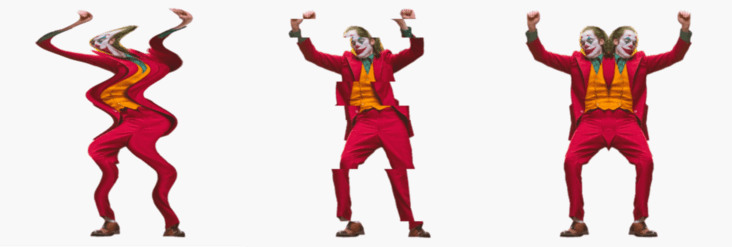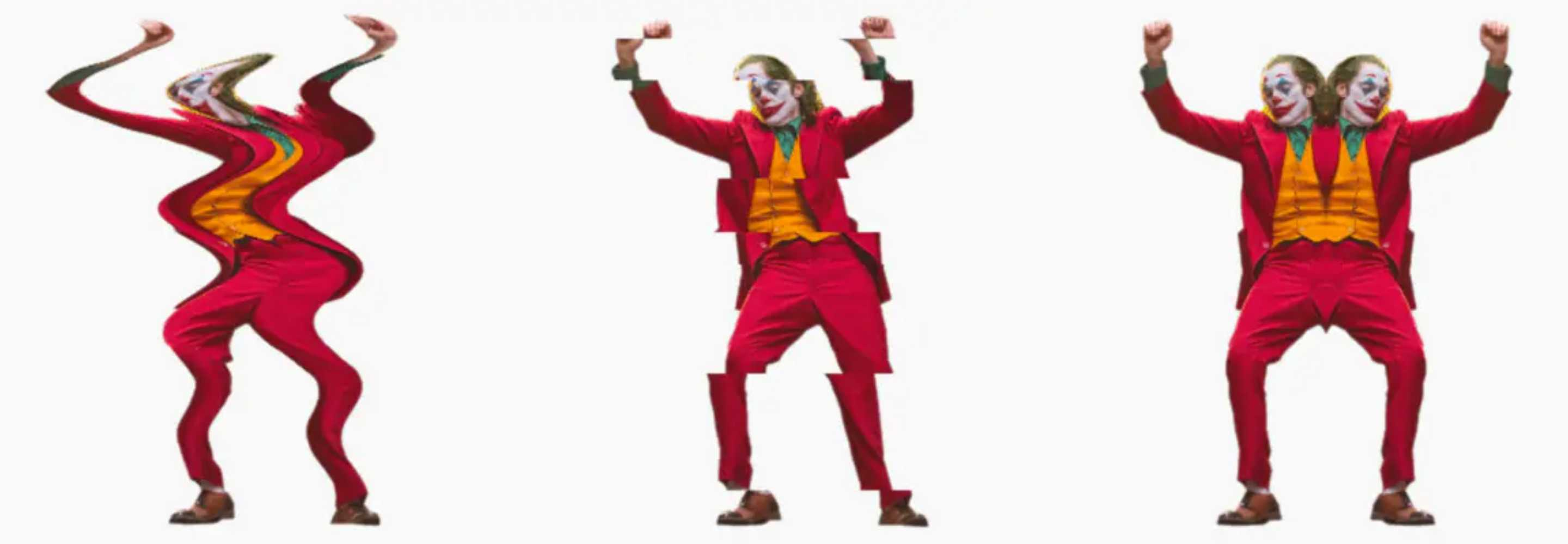# 后处理——深入相机变形特效

### 概述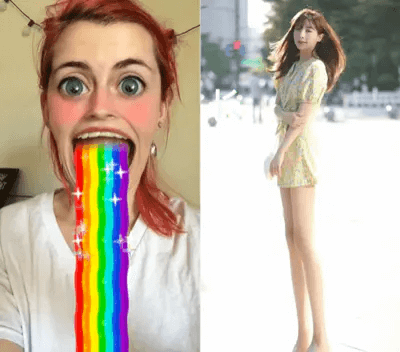• 局部扭曲（twirl effect）
• 局部膨胀（inflate effect）
• 任意方向挤压（pinch effect）

### 变形技原理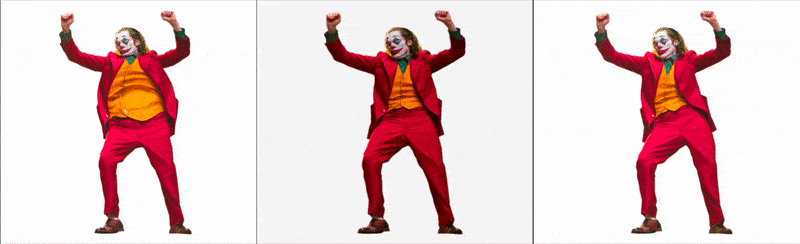``````#iChannel0 "src/assets/texture/joker.png"

vec2 deform(vec2 uv, vec2 center, float range, float strength) {
// TODO: 变形处理
return uv;
}

void mainImage(out vec4 fragColor, vec2 coord) {
vec2 uv = coord / iResolution.xy;
vec2 mouse = iMouse.xy / iResolution.xy;
uv = deform(uv, mouse, .5, .5);
vec3 color = texture(iChannel0, uv).rgb;
fragColor.rgb = color;
}
``````

### 变形小技巧：采样距离场变换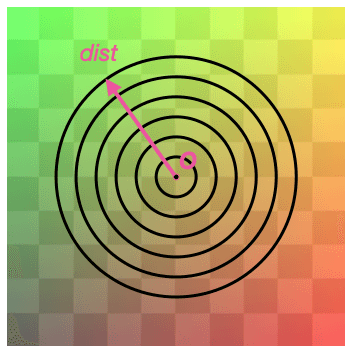``````vec2 deform(vec2 uv, vec2 center, float range, float strength) {
float dist = distance(uv, center);
vec2 direction = normalize(uv - center);
dist = transform(dist, range, strength); // 改变采样圈半径
center = transform(center, dist, range, strength); // 改变采样圈中心位置
return center + dist * direction;
}
``````

### 扭曲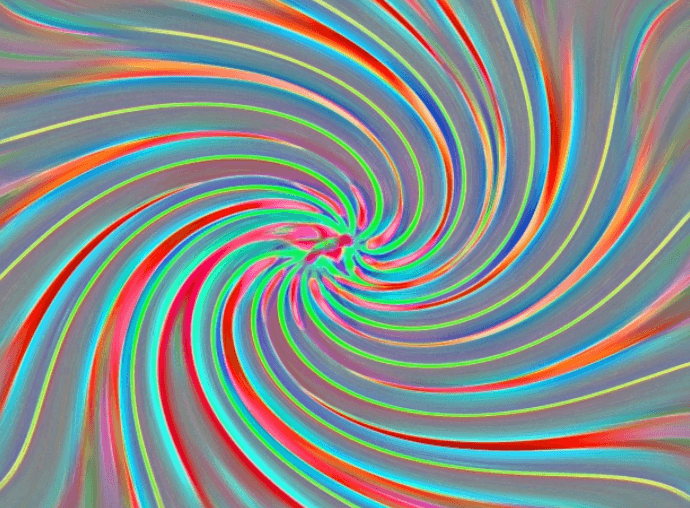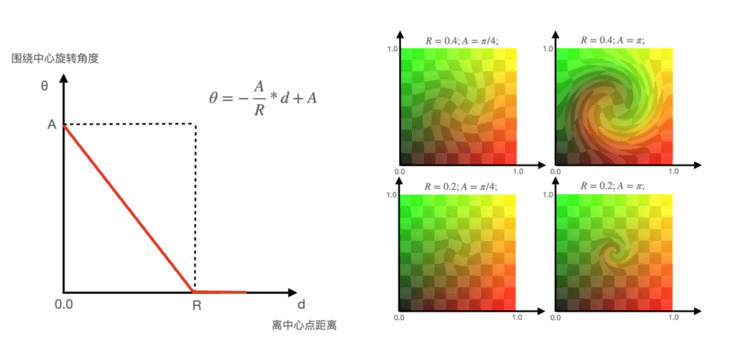1）A代表中心旋转角度，绝对值越大，扭曲程度更高；
2）A>0表示扭曲方向为顺时针，反之A<0表示逆时针；
3）R代表扭曲边界，值越大，影响范围越大。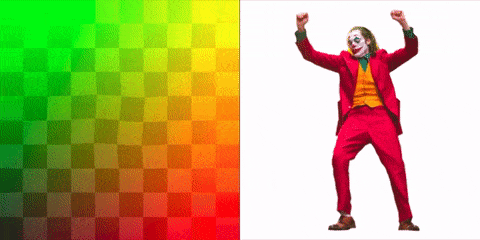``````#iChannel0 "src/assets/texture/joker.png"
#define Range .3
#define Angle .5
#define SPEED 3.
mat2 rotate(float a) // 旋转矩阵
{
float s = sin(a);
float c = cos(a);
return mat2(c,-s,s,c);
}
vec2 twirl(vec2 uv, vec2 center, float range, float angle) {
float d = distance(uv, center);
uv -=center;
// d = clamp(-angle/range * d + angle,0.,angle); // 线性方程
d = smoothstep(0., range, range-d) * angle;
uv *= rotate(d);
uv+=center;
return uv;
}
void mainImage(out vec4 fragColor, vec2 coord) {
vec2 uv = coord / iResolution.xy;
vec2 mouse = iMouse.xy / iResolution.xy;
float cTime = sin(iTime * SPEED);
uv = twirl(uv, mouse, Range, Angle * cTime);
vec4 color = texture(iChannel0, uv);
fragColor = color;
}
``````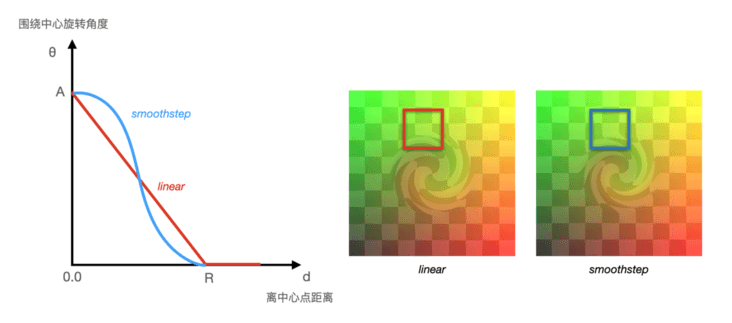linear和smoothstep扭曲方程效果对比

### 膨胀/收缩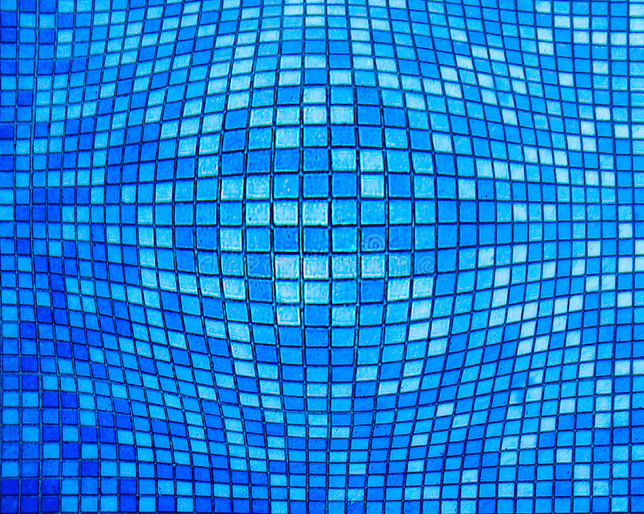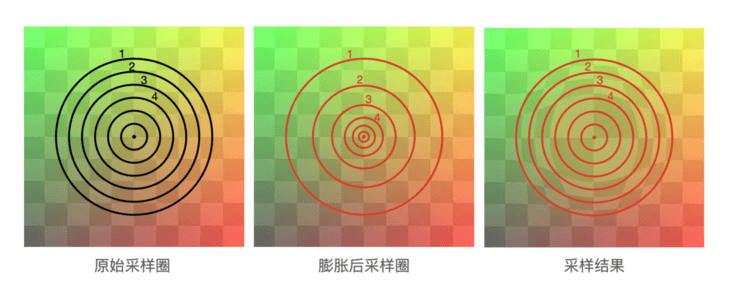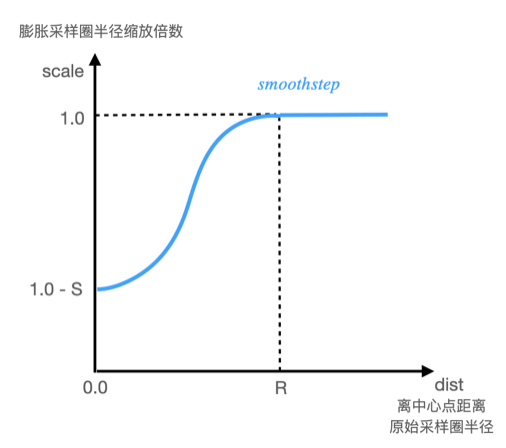``````float scale = (1.- S) + S * smoothstep(0.,1., dist / R); // 计算膨胀采样半径缩放值
``````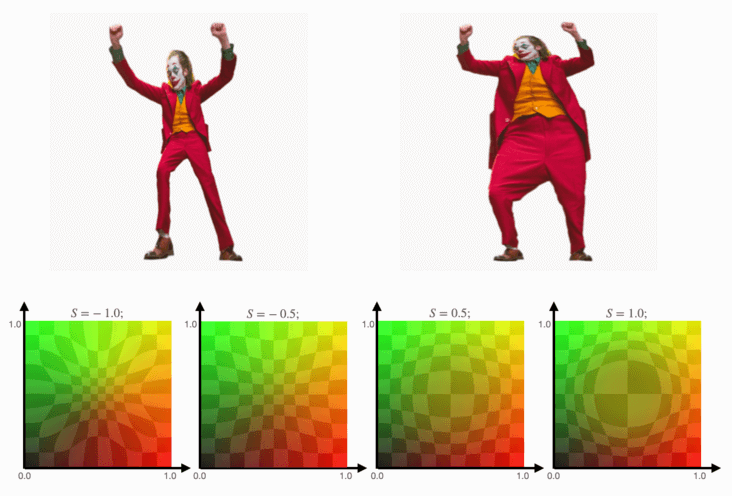S值对应膨胀收缩程度Strength

1）当S在[0,1]区间时，呈现膨胀效果，S值越大，膨胀的程度越高；
2）当S在[-10]区间时，呈现收缩效果，S值越小，收缩程度越高；
3）R代表变形的边界，值越大时，影响区域越大；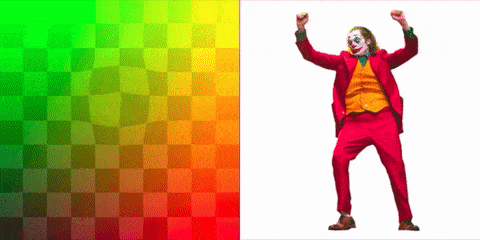``````#iChannel0 "src/assets/texture/joker.png"
#define SPEED 2. // 速度
#define RANGE .2 // 变形范围
#define Strength .5 * sin(iTime * SPEED) // 变形程度

vec2 inflate(vec2 uv, vec2 center, float range, float strength) {
float dist = distance(uv , center);
vec2 dir = normalize(uv - center);
float scale = 1.-strength + strength * smoothstep(0., 1. ,dist / range);
float newDist = dist * scale;
return center + newDist * dir;
}
void mainImage(out vec4 fragColor, vec2 coord) {
vec2 uv = coord / iResolution.xy;
vec2 mouse = iMouse.xy / iResolution.xy;
uv = inflate(uv, mouse, RANGE, Strength);
vec3 color = texture(iChannel0, uv).rgb;
fragColor.rgb = color;
}
``````

### 纵向/横向拉伸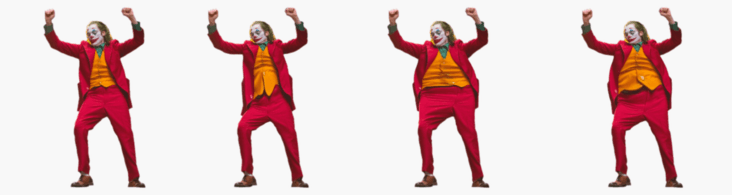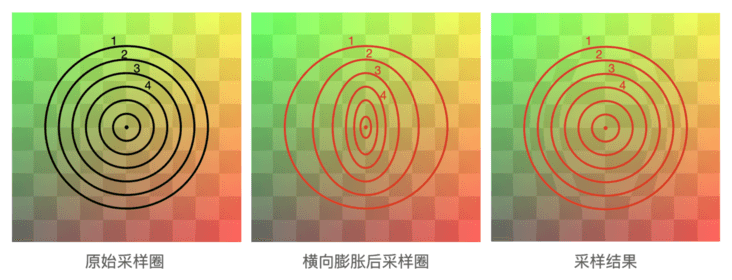``````vec2 inflateX(vec2 uv, vec2 center, float radius, float strength) {
// 前面代码跟膨胀实现一样
...
return center + vec2(newDist, dist) * dir; // 横向拉伸则scale只作用于想x轴
}
``````

### 挤压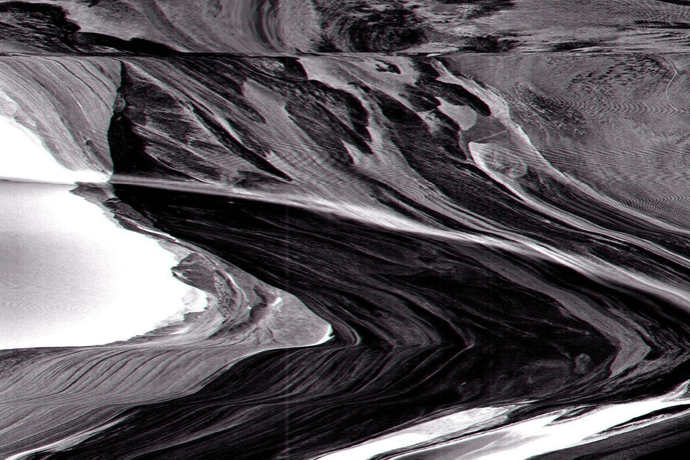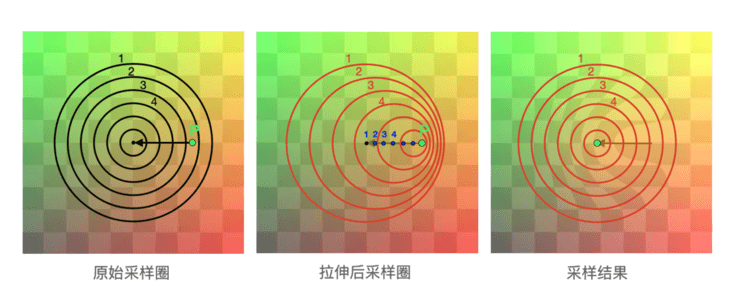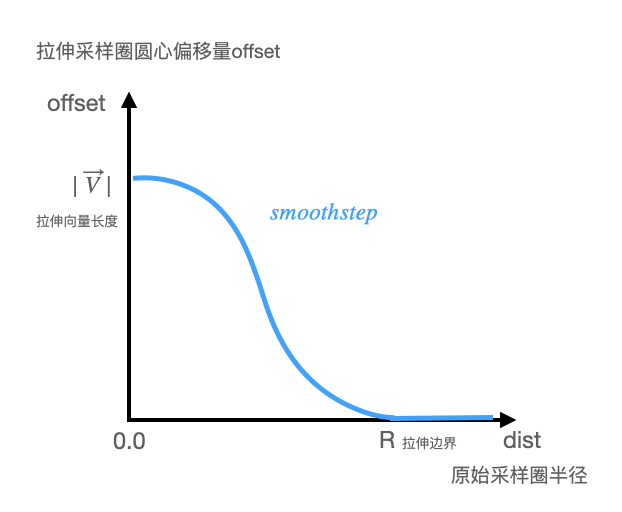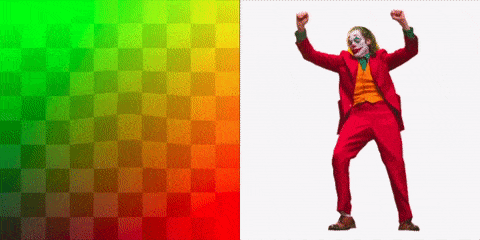``````#iChannel0 "src/assets/texture/joker.png"
#define RANGE .25  // 变形范围
#define PINCH_VECTOR vec2( sin(iTime * 10.), cos(iTime * 20.)) * .03 // 挤压向量

vec2 pinch(vec2 uv, vec2 targetPoint, vec2 vector, float range)
{
vec2 center = targetPoint + vector;
float dist = distance(uv, targetPoint);
vec2 point = targetPoint +  smoothstep(0., 1., dist / range) * vector;
return uv - center + point;
}
void mainImage(out vec4 fragColor, vec2 coord) {
vec2 uv = coord / iResolution.xy;
vec2 mouse = iMouse.xy / iResolution.xy;
uv = pinch(uv, mouse, PINCH_VECTOR, RANGE);
vec3 color = texture(iChannel0, uv).rgb;
fragColor.rgb = color;
}
``````

### 总结